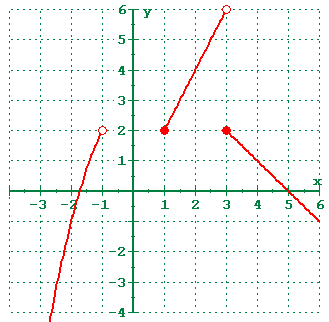# Domain notation

Shaybay92
Just wondering how to write the domain of where a function similar to this is continuous:using proper domain notation.

KrisOhn
For stating a domain or range of a function, I'm pretty sure its accepted to use interval notation.
http://en.wikipedia.org/wiki/Interval_(mathematics)

And if a function is entirely continuous, I think it could be simply stated as

$$x| \Re$$
(x is continuous over the set of all real numbers)

Studiot
Do you mean continuous or connected?
Your 'function', if it's the set of red lines' is not connected.

For stating a domain or range of a function, I'm pretty sure its accepted to use interval notation.
http://en.wikipedia.org/wiki/Interval_(mathematics)
This makes no sense at all. The domain or range of a function need not be an interval.

The domain of a function is just a set. So any proper way to denote a set is a proper way to denote the domain.

Homework Helper
Yes, but the set of points at which this particular function is continuous ("domain" is the wrong word) is the union of three intervals. Using interval notation to state that set is perfectly correct.

Since Shaybay92 said, in the original post, that interval notation was to be used in stating the answer, I have no idea what KrisOhn meant by saying " I'm pretty sure its accepted to use interval notation." Neither that nor his Wikipedia link to what an interval is contributes anything to the problem of determining what those intervals are.

Shaybay92, you should be able to see from the graph that this function is continuous on three intervals. The set can be written as the union of those three intervals:
$$(-\infty, a]\cup [b, c)\cup [d, \infty)$$

You should be able to see from the graph what a, b, c, and d are.

Last edited by a moderator:
Of course it is correct, but it's rather tautological: if a set you wish to denote is an interval, then writing down that interval is a correct way to do it.

But KrisOhn did not even refer to this particular function, which happens to have as domain (and set of points where it's continous) the union of intervals. He said that to denote the domain of an arbitrary function (well, he said "a function"), interval notation is used. This is obviously false.

Studiot
Assuming HOI has the correct interpretation of the question, and that an open circle means an open interval and a filled in circle means a closed endpoint
I think only the first interval is continuous.

Is the second interval not discontinuous at b and the third at d?

An interval cannot be continuous, a function can be continuous on (points in) an interval.

The function is not continuous at -1, since -1 is not even in the domain.
The function is continuous at 1, since the limit as x approaches 1 is the same as the right limit; there is no left limit because there are no domain points to the left of 1 (sloppy language, but consider small open balls around x=1).
The function is not continuous at 3, because left and right limits do not coincide.

So the desired set is $$(-\infty,-1)\cup[1,3)\cup(3,\infty)$$.
This is the same as $$D\backslash\{3\}$$, where D is the domain of the function.

Studiot
Yes some sloppy Sunday terminology all round I whit.

But collectively we got there in the end.

I think the last offering by Landau to be correct.